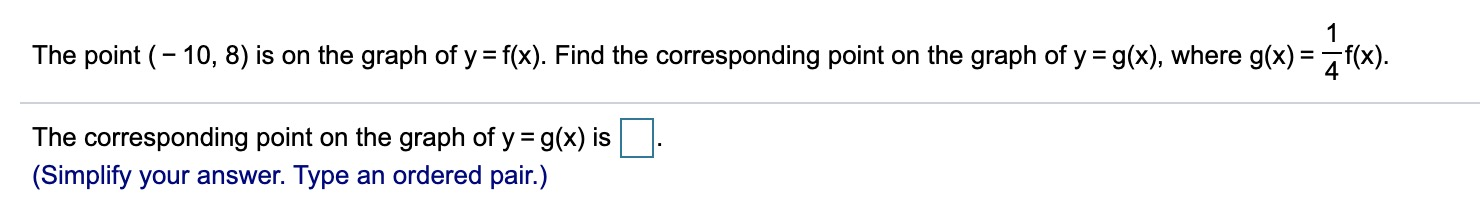# 1The point (10, 8) is on the graph of yf(x). Find the corresponding point on the graph of y g(x), where g(x) f(x)The corresponding point on the graph of y g(x) is(Simplify your answer. Type an ordered pair.)

Question
3 views

The point (−10​,8​) is on the graph of y=​f(x). Find the corresponding point on the graph of y=g(x), where g(x)=1/4 f(x)help_outlineImage Transcriptionclose1 The point (10, 8) is on the graph of y f(x). Find the corresponding point on the graph of y g(x), where g(x) f(x) The corresponding point on the graph of y g(x) is (Simplify your answer. Type an ordered pair.) fullscreen
check_circle

Step 1

It is given that the point (-10,8) is on the graph of the function

Step 2

In order to determine the corresponding point on the graph of the function,

Step 3

Divide the function by 4. Therefore, divide the y value of the given ...

### Want to see the full answer?

See Solution

#### Want to see this answer and more?

Solutions are written by subject experts who are available 24/7. Questions are typically answered within 1 hour.*

See Solution
*Response times may vary by subject and question.
Tagged in

### Other